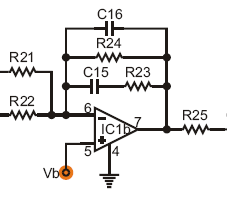###Author Topic: A theory/calculation question about emphasis circuits  (Read 1458 times)

#### Mark Hammer

• more
• Poster2
•• Posts: 26110
• Total likes: 2004##### A theory/calculation question about emphasis circuits
« on: January 31, 2008, 03:12:14 PM »
If you've looked at things like the Boss DM-2 and CE-2/3, etc., it is a common practice to include treble pre-emphasis and de-emphasis as a kind of "poorman's DolbyTM".  The assumption is that if you apply treble emphasis and a corresponding identical de-emphasis to the dry signal, you end up with the same tonal balance you started with, but if you apply the treble de-emphasis to the delay signal only, you reduce any hiss acquired via the delay path.

When the pre-emphasis is part of an inverting stage, where I can easily calculate the extra boost provided to the top end, one can make an easy inference about the corresponding de-emphasis at the other end.  So, looking at the schematic for the Boss CE-2 (see the Tonepad Corrral layout here: http://www.tonepad.com/getFile.asp?id=101), you can see that C3/R5 bypass R4, providing a more "efficient" path for treble content.  With R5=10k and the feedback resistance (R6) = 47k, there is a gain of 1x applied full-band, but a gain of 5 applied to content above 1 / [2*pi*C*R].  In this case, that is content above roughly 2340hz.

Where I have trouble is in working backwards when I don't have the pre-emphasis calculations to guide me.  That is, when I only see the de-emphasis.  More specifically, if one's intention was simply to provide a rolloff gentler than the 6db/oct that comes with the traditional feedback resistor and cap in parallel, how the dickens would you calculate it, and how would you know precisely how many db/oct a slope you had?

Here's an illustration, taken from the Tonepad CE-2 schematic (thanks again Francisco!), the output mixer stage specifically.  C16=100pf, R24=47k, R23=10k, C15 = .0068uf.  The 100pf/47k pair gives me a rolloff above 33khz, so I won't factor that into my audible calculations.  If R23 were 47k, there would clearly be no advantage for any HF content travelling along that path.  After all, the other path is also 47k.  So, the effect of C15 on treble rolloff will really only start to kick in as R23 becomes less than R24; correct?  And, if I have understood things correctly, the actual rolloff point (corner frequency or "knee") is recalculated, based on the joint product of R23/C15.

Following from this, if one wanted to have a rolloff that started from roughly the same point but was "gentler", you could use, say, a 39k value for R23 instead of 10k and a .0018uf cap for C15 ( 1/[2*pi*C*R] = 2267hz )?   So, what would the calculated slope of that rolloff be?#### alanlan

• Poster2
•• Posts: 531
• Total likes: 0##### Re: A theory/calculation question about emphasis circuits
« Reply #1 on: January 31, 2008, 06:43:43 PM »
Ignoring the 100pF because that's there to maintain HF stability.

The remaining circuit is a shelving filter i.e. at low frequency, gain is determined by R24 (and the input Rs), and at some higher frequency it is determined by R24//R23.  It's first order, so if R23 were much less in value than R24, there would be a 6dB/octave roll-off between the two gains.  If R24 is not so much less, i.e. the low and high frequency gains aren't that much different, then the slope never quite makes it to 6dB/octave before it flattens out to the high frequency gain.

#### alanlan

• Poster2
•• Posts: 531
• Total likes: 0##### Re: A theory/calculation question about emphasis circuits
« Reply #2 on: January 31, 2008, 06:57:04 PM »

The roll off frequency (pole) i.e. the corner frequency where it starts to roll off is:
1/(2*PI*C15.(R24+R23))

The frequency where it flattens out (zero) is given by:
1/(2*PI*C15.R23)

Note that there isn't necessarily a 3dB point, because the depth of the shelf may be less than 3dB.

#### alanlan

• Poster2
•• Posts: 531
• Total likes: 0##### Re: A theory/calculation question about emphasis circuits
« Reply #3 on: February 01, 2008, 08:24:29 AM »
Something interesting I realised some time ago about first order shelving filters.  (OK it's perhaps not that interesting)

If you think about the ubiquitous 6dB/octave slope which is 20dB per decade,  it is a decade in gain (X10 or 1/10) for each decade in frequency so the slope has a gradient of 1.

Interpreted another way, the ratio f2/f1 is equal to the ratio of gain2/gain1 (or gain1/gain2 depending on whether the "shelf" is boosting or cutting).

You cannot get away from this, it is fixed.  So if you want a lot of cut or boost in the shelf, then the frequencies where the shelf starts and ends will be further apart.  Similarly, if you want the shelf corners to be further apart, then the shelf will be deeper in terms of gain or attenuation.

If you visualise the shelf slope as always being 1 (which it is asymptotically), and then visualise the shelf becoming deeper, you should see what I mean.

I hope this all makes sense.  (A drawing would have been useful, I know).

#### gaussmarkov##### Re: A theory/calculation question about emphasis circuits
« Reply #4 on: February 01, 2008, 02:32:57 PM »
thanks for pointing this out.  i had not caught on to the "maximum slope" phenomenon yet.gaussmarkov.net:  layouts & eagle